# Nitric Acid.pdf

1. Jun 2023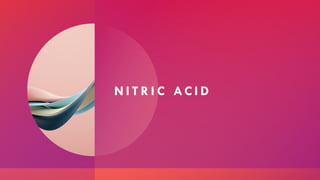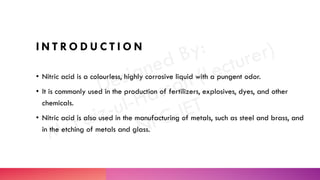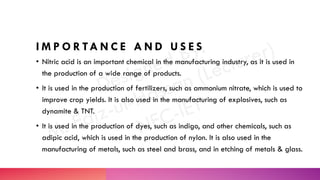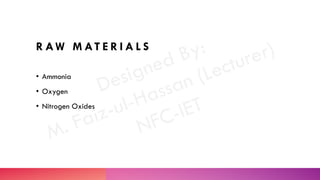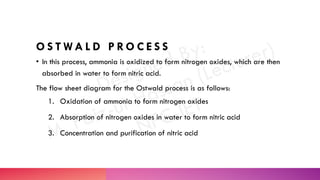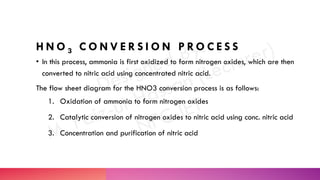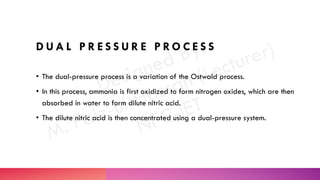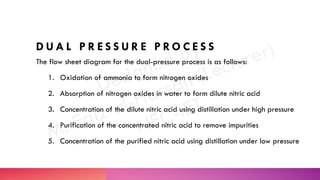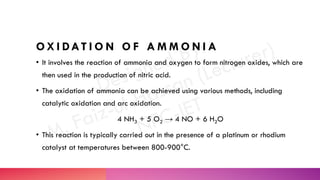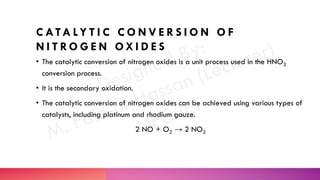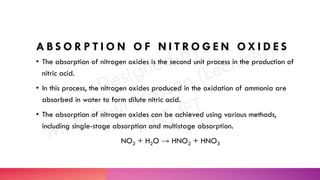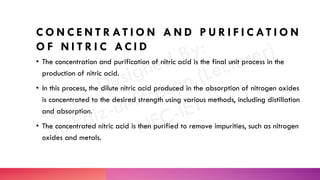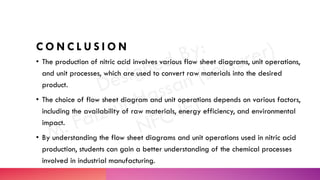1 von 16

### Nitric Acid.pdf

• 1. N I T R I C AC I D
• 2. I N T R O D U C T I O N • Nitric acid is a colourless, highly corrosive liquid with a pungent odor. • It is commonly used in the production of fertilizers, explosives, dyes, and other chemicals. • Nitric acid is also used in the manufacturing of metals, such as steel and brass, and in the etching of metals and glass.
• 3. I M P O R TA N C E A N D U S E S • Nitric acid is an important chemical in the manufacturing industry, as it is used in the production of a wide range of products. • It is used in the production of fertilizers, such as ammonium nitrate, which is used to improve crop yields. It is also used in the manufacturing of explosives, such as dynamite & TNT. • It is used in the production of dyes, such as indigo, and other chemicals, such as adipic acid, which is used in the production of nylon. It is also used in the manufacturing of metals, such as steel and brass, and in etching of metals & glass.
• 4. R AW M AT E R I A L S • Ammonia • Oxygen • Nitrogen Oxides
• 5. F L O W S H E E T D I AG R A M S
• 6. O S T W A L D P R O C E S S • In this process, ammonia is oxidized to form nitrogen oxides, which are then absorbed in water to form nitric acid. The flow sheet diagram for the Ostwald process is as follows: 1. Oxidation of ammonia to form nitrogen oxides 2. Absorption of nitrogen oxides in water to form nitric acid 3. Concentration and purification of nitric acid
• 7. H N O 3 C O N V E R S I O N P R O C E S S • In this process, ammonia is first oxidized to form nitrogen oxides, which are then converted to nitric acid using concentrated nitric acid. The flow sheet diagram for the HNO3 conversion process is as follows: 1. Oxidation of ammonia to form nitrogen oxides 2. Catalytic conversion of nitrogen oxides to nitric acid using conc. nitric acid 3. Concentration and purification of nitric acid
• 8. D U A L P R E S S U R E P R O C E S S • The dual-pressure process is a variation of the Ostwald process. • In this process, ammonia is first oxidized to form nitrogen oxides, which are then absorbed in water to form dilute nitric acid. • The dilute nitric acid is then concentrated using a dual-pressure system.
• 9. D U A L P R E S S U R E P R O C E S S The flow sheet diagram for the dual-pressure process is as follows: 1. Oxidation of ammonia to form nitrogen oxides 2. Absorption of nitrogen oxides in water to form dilute nitric acid 3. Concentration of the dilute nitric acid using distillation under high pressure 4. Purification of the concentrated nitric acid to remove impurities 5. Concentration of the purified nitric acid using distillation under low pressure
• 10. U N I T O P E R AT I O N S & U N I T P RO C E S S E S
• 11. O X I DAT I O N O F A M M O N I A • It involves the reaction of ammonia and oxygen to form nitrogen oxides, which are then used in the production of nitric acid. • The oxidation of ammonia can be achieved using various methods, including catalytic oxidation and arc oxidation. 4 NH3 + 5 O2 → 4 NO + 6 H2O • This reaction is typically carried out in the presence of a platinum or rhodium catalyst at temperatures between 800-900°C.
• 12. C ATA LY T I C C O N V E R S I O N O F N I T R O G E N O X I D E S • The catalytic conversion of nitrogen oxides is a unit process used in the HNO3 conversion process. • It is the secondary oxidation. • The catalytic conversion of nitrogen oxides can be achieved using various types of catalysts, including platinum and rhodium gauze. 2 NO + O2 → 2 NO2
• 13. A B S O R P T I O N O F N I T R O G E N O X I D E S • The absorption of nitrogen oxides is the second unit process in the production of nitric acid. • In this process, the nitrogen oxides produced in the oxidation of ammonia are absorbed in water to form dilute nitric acid. • The absorption of nitrogen oxides can be achieved using various methods, including single-stage absorption and multistage absorption. NO2 + H2O → HNO2 + HNO3
• 14. C O N C E N T R AT I O N A N D P U R I F I C AT I O N O F N I T R I C AC I D • The concentration and purification of nitric acid is the final unit process in the production of nitric acid. • In this process, the dilute nitric acid produced in the absorption of nitrogen oxides is concentrated to the desired strength using various methods, including distillation and absorption. • The concentrated nitric acid is then purified to remove impurities, such as nitrogen oxides and metals.
• 15. C O N C L U S I O N
• 16. C O N C L U S I O N • The production of nitric acid involves various flow sheet diagrams, unit operations, and unit processes, which are used to convert raw materials into the desired product. • The choice of flow sheet diagram and unit operations depends on various factors, including the availability of raw materials, energy efficiency, and environmental impact. • By understanding the flow sheet diagrams and unit operations used in nitric acid production, students can gain a better understanding of the chemical processes involved in industrial manufacturing.